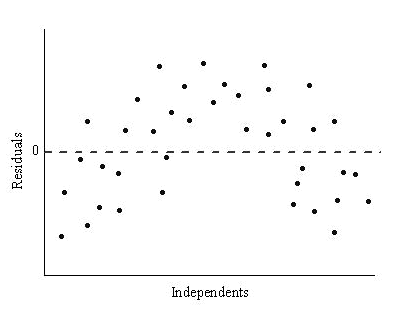# AP Statistics : How to do logarithmic transformations

## Example Questions

Explanation:

### Example Question #19 : Bivariate Data

What transformation should be done to the data set, with its residual shown below, to linearize the data?multiply the independent variable byAddto the y-value of each data point

multiply the dependent variable by a constant k.

take the log of the dependent variable

Nothing, the data set is already linear